|

# 【壹家壹品】全新品牌升级,定制家具黑马描绘新篇章!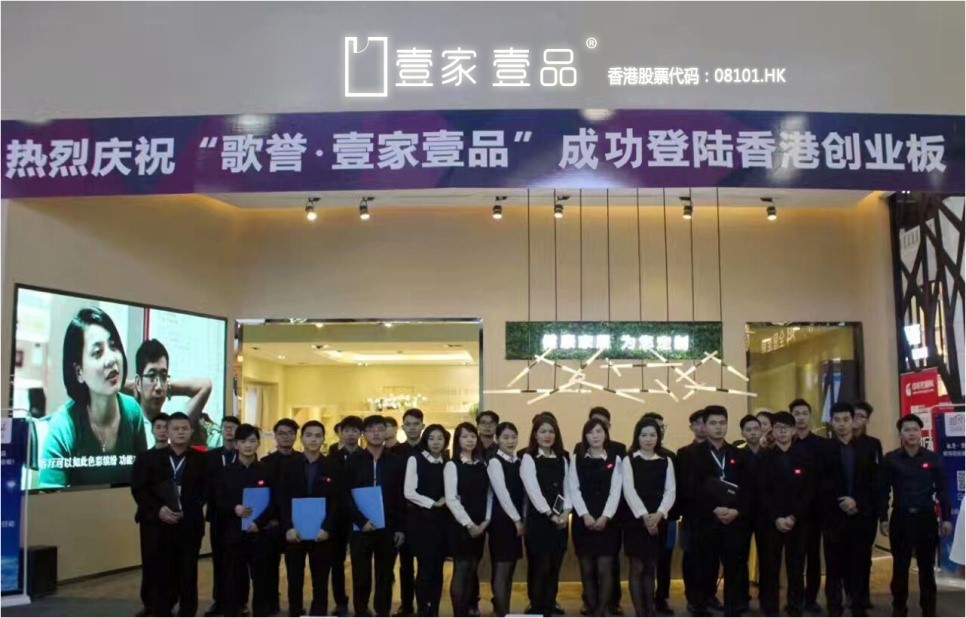壹家壹品，自2012年创立以来发展迅猛，无论在产品研发、产品生产、终端营销还是内部运营上，均取得突破性成果，成长速度之快居行业前列！2017年更是成功登录香港创业板，香港股票代码08101.HK，创立5年不到即成功上市，一举刷新上市同行时间记录，成为实至名归的“黑马”！

目前为止，壹家壹品全新品牌升级工作已基本完成，下面就和各位全方位解读一下其品牌升级后的新内涵。

一、全新品牌定位，体现长远战略考量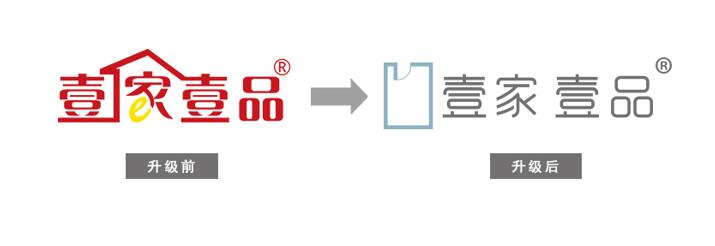（上图为新旧logo对比）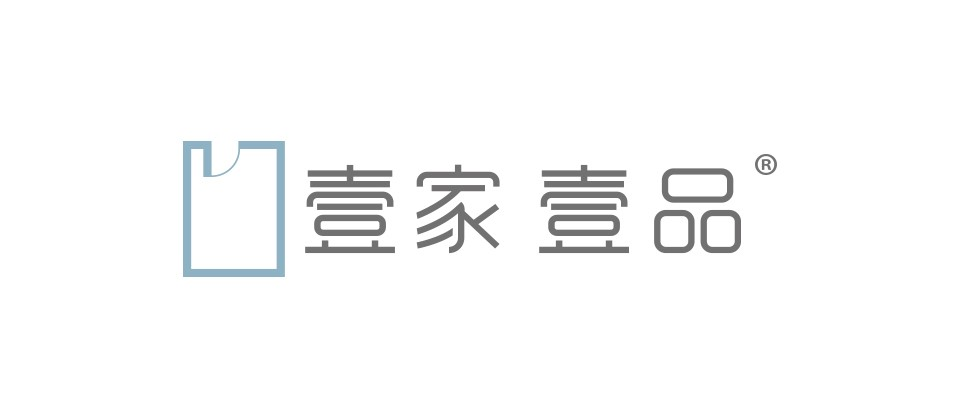（上图为新logo特写图）

随着80、90后逐渐步入成家立业的阶段，为了契合新一代消费群体审美观念的变迁，经过深入的市场调研，壹家壹品将全新的品牌定位定义为：

为追求“个性时尚”的年轻中产阶层，量身设计彰显个人风格及品味的优质家居空间！

新的品牌定位体现了壹家壹品长远的战略考量，此次的品牌新升级以及以后的产品研发、活动策划等，都会紧密围绕此品牌定位展开，务求形成统一鲜明的品牌风格和品牌标识。

二、全新品牌logo，重新定义“家”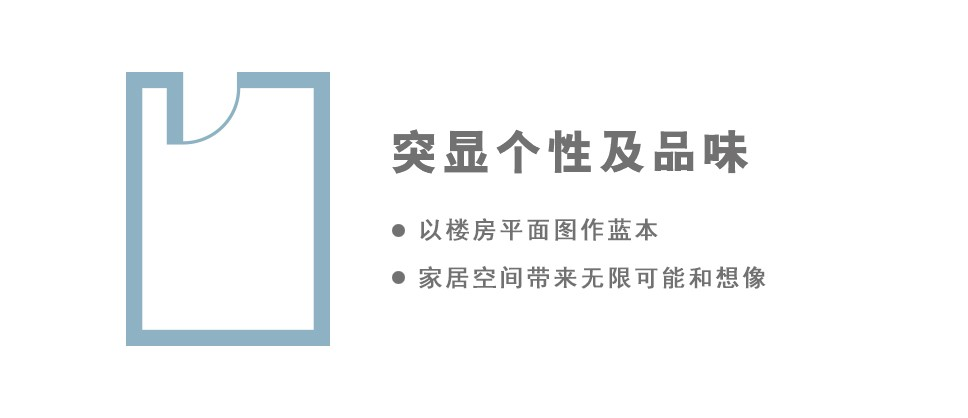新品牌logo含义：全新设计的壹家壹品logo，以楼房平面图作蓝本，寓意壹家壹品能为家居空间带来无限可能和想像。

三、新品牌口号，我的家居新主张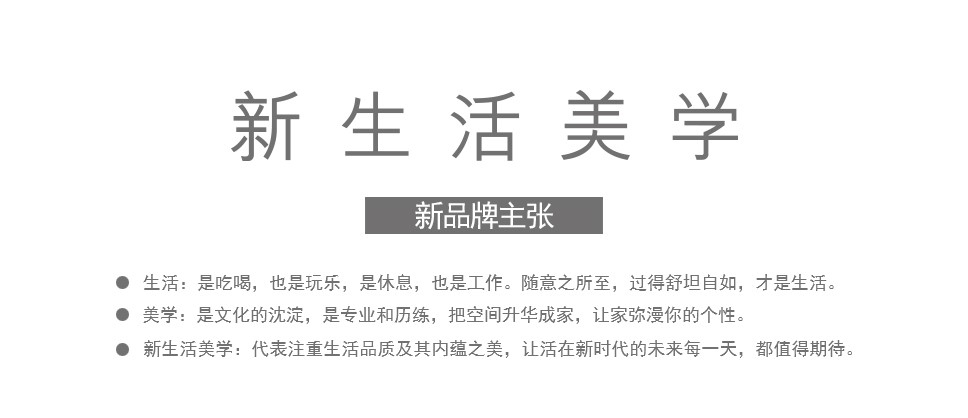“新生活美学”——壹家壹品的新品牌口号，亦即品牌主张，涵盖了壹家壹品立足当下，放眼未来的核心价值。

新，是另觅蹊径，

与别不同，是每天都有新意，和新鲜感。

生活，是吃喝，也是玩乐，是休息，

也是工作。随意之所至，过得舒坦自如，才是生活。

美学，是文化的沉淀，是专业和历练，把空间升华成家，

让家弥漫你的个性。

新生活美学，代表注重生活品质及其内蕴之美，让活在新时代的未来每一天，都值得期待。

四、新品牌英文名，迈向国际化第一步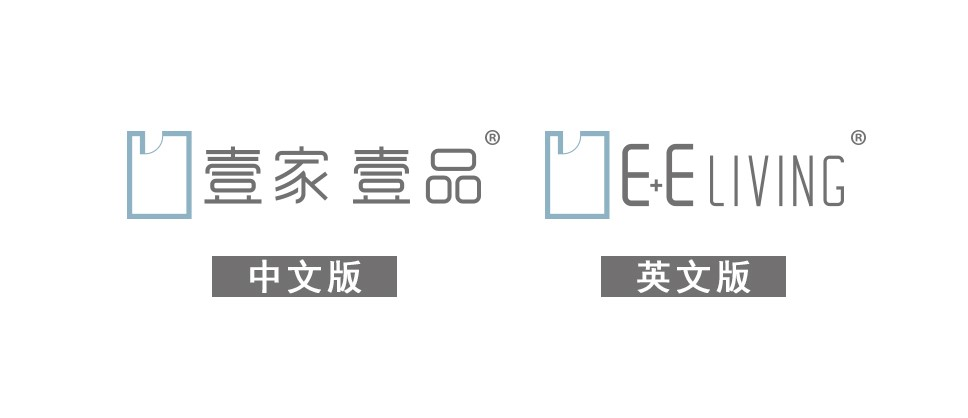上面为壹家壹品中文版logo和英文版logo的对比，可以看到它们的关联度和辨识度都非常高。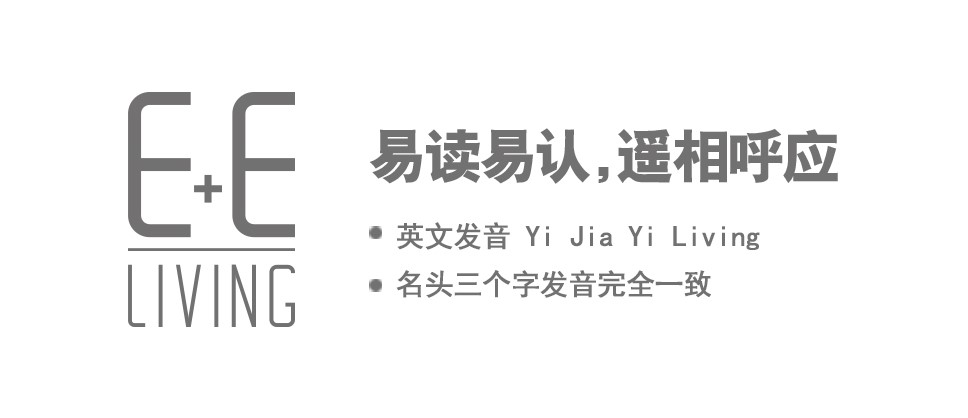E + E Living是壹家壹品的新品牌英文名，英文发音 Yi Jia Yi Living，和中文名头三个字发音完全一致，而且拼写简单，易读易认，有助加深大众对品牌的印象，而且E + E Living这个英文名非常有“国际范”，彰显了壹家壹品面向国际市场的决心。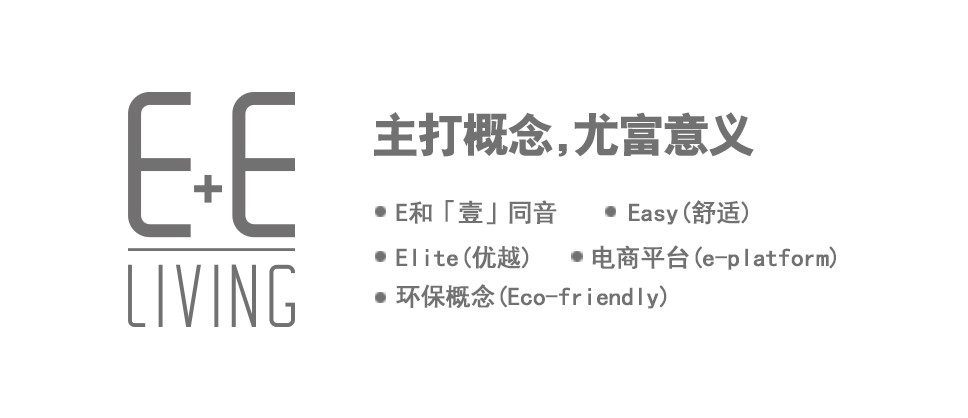不仅如此，E，和「壹」同音，是 “Easy” (舒适) 和“Elite” (优越) 的首个字母，刚好与「壹家」和「壹品」遥相呼应。

此外，品牌同时主打的环保概念 (Eco-friendly)及电商平台 (e-platform)，英文皆以“E”开首，选用 “E+E Living”, 尤富意义。

五，全新旗舰店设计，体验感新升级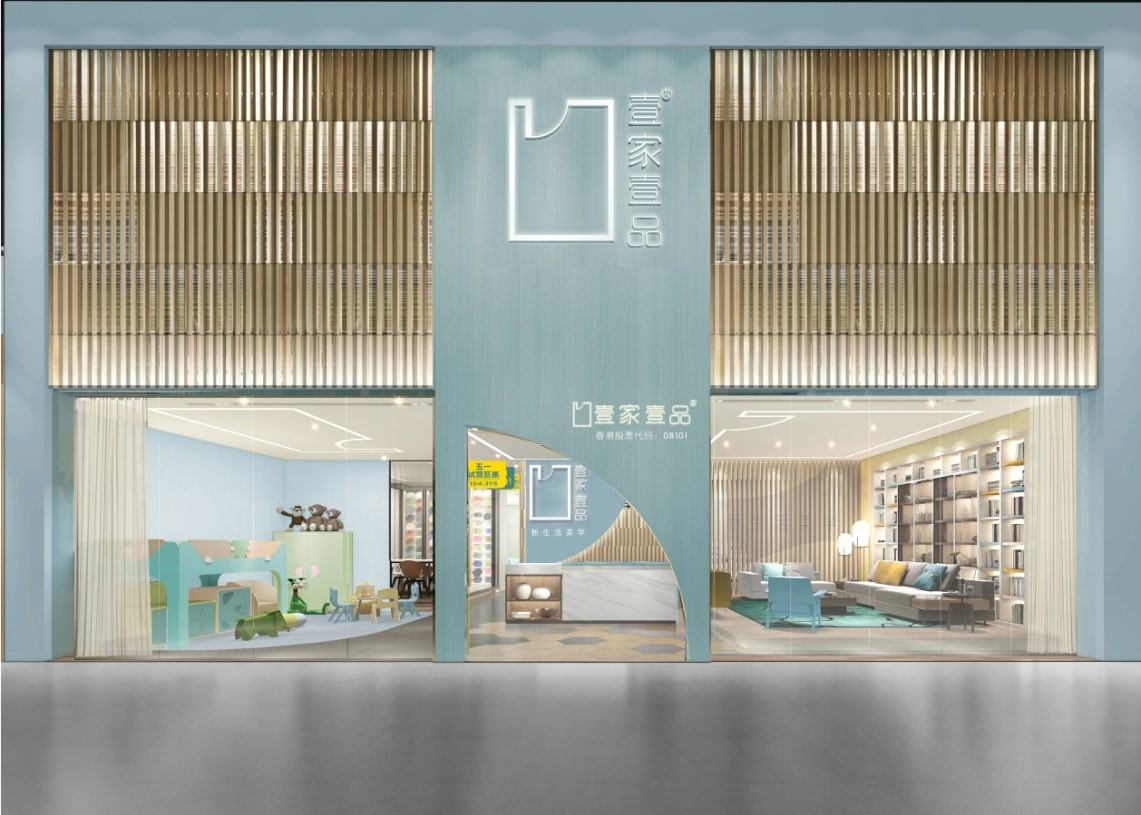（图为旗舰店的门头设计）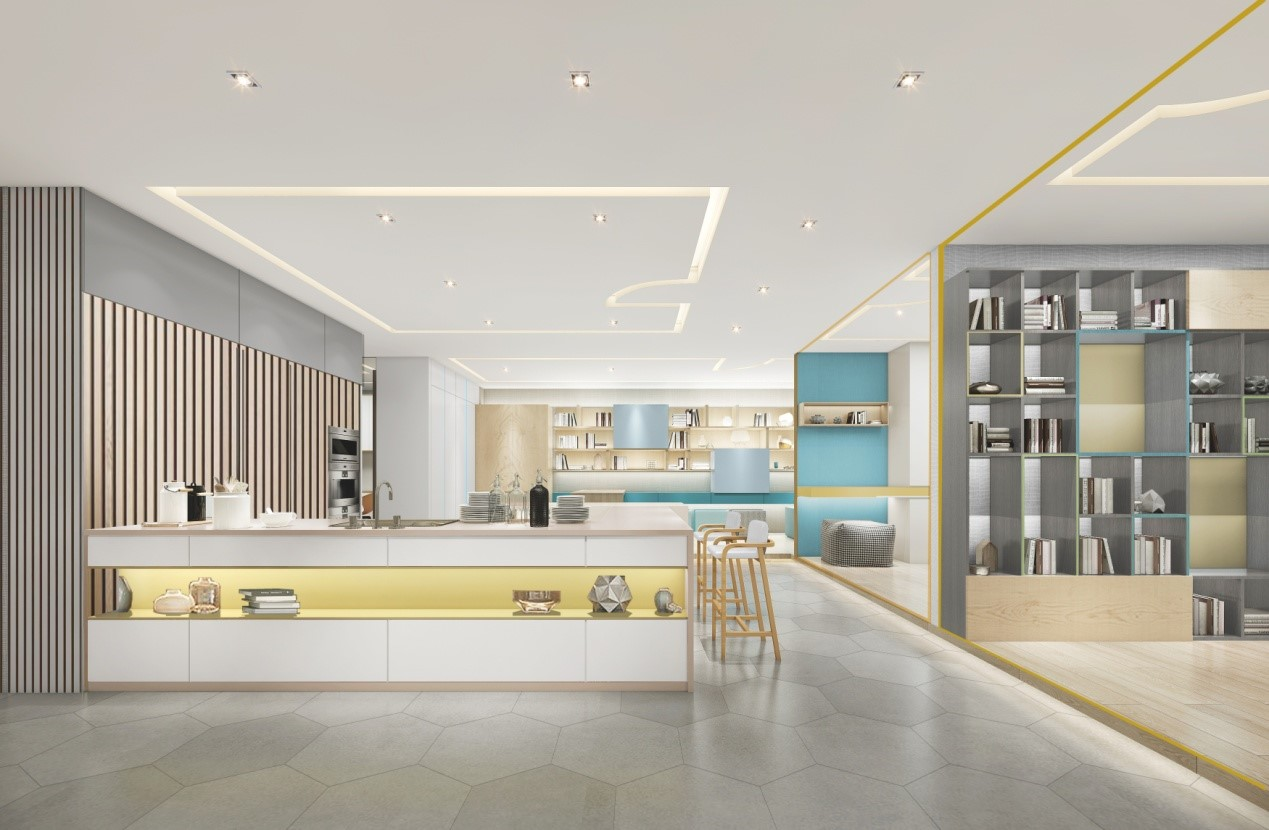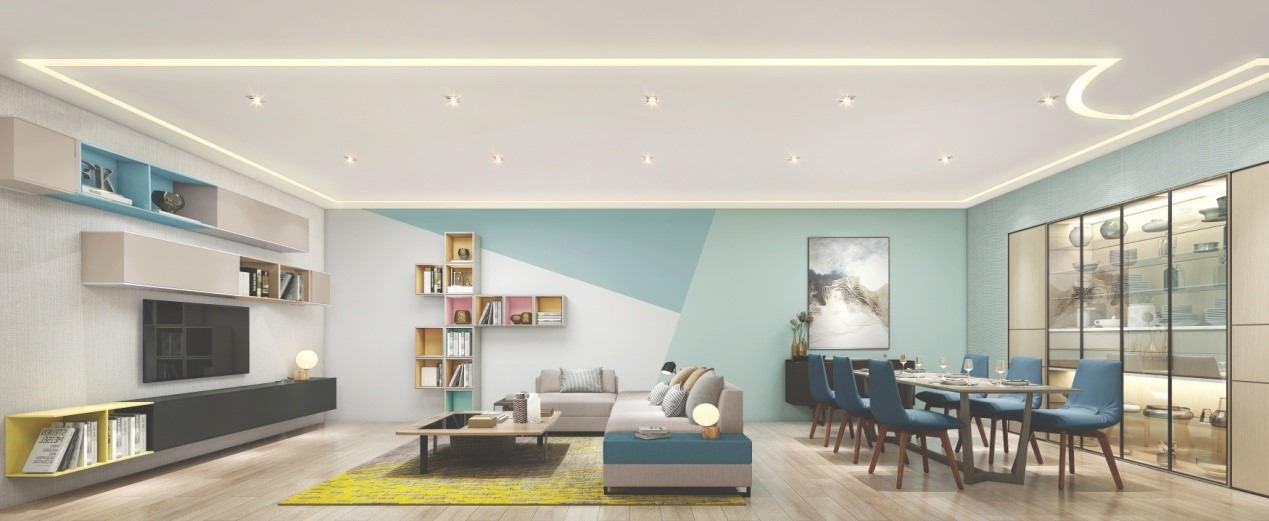（上面三图为旗舰店的内部设计）

这次壹家壹品全新品牌升级专门聘请了香港知名的KS设计事务所来负责新的旗舰店设计，旗舰店的整体风格将更具艺术感，里面包含卧房、客厅、儿童房、长辈房、洽谈区、设计师设计区等不同区域的展示，并采用新的设计语言，风格偏简约、时尚、温暖，更符合新一代消费群体的口味。

六，从化新展厅，完整呈现全新品牌形象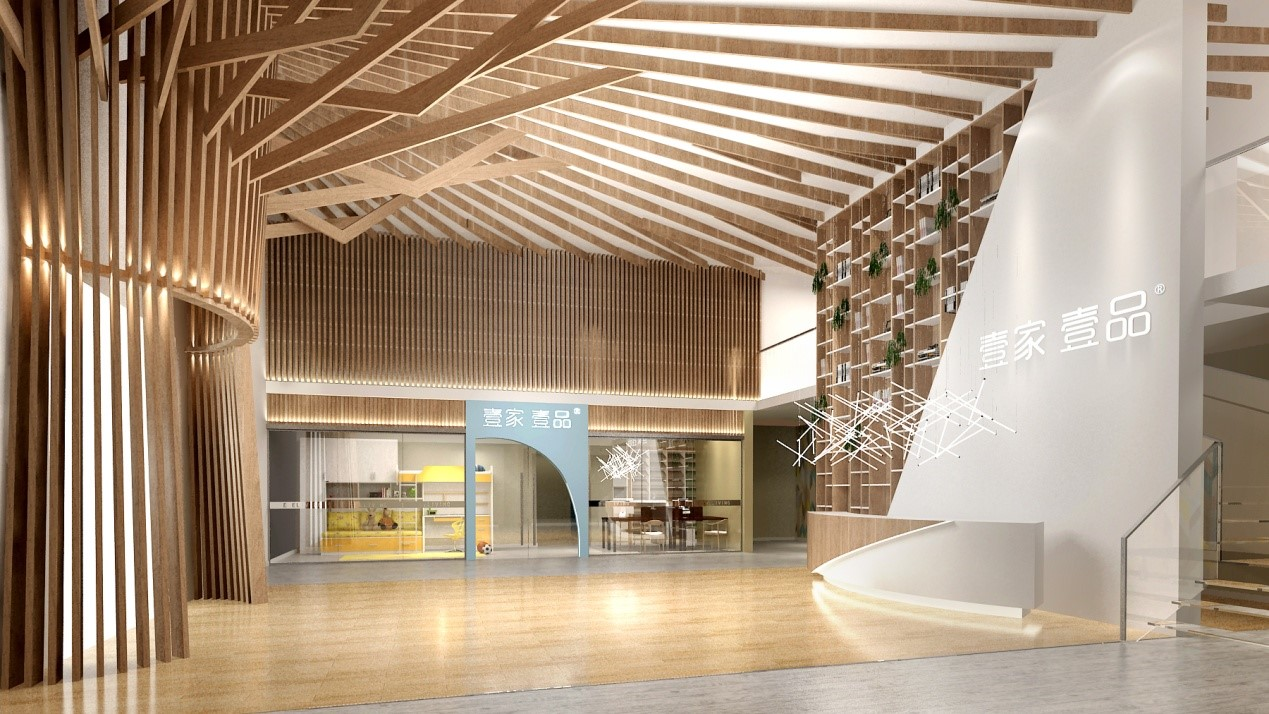（上图为壹家壹品从化展厅大堂）

为了系统、完整地呈现壹家壹品的全新品牌形象，我们将在壹家壹品的从化十万平米工业园区内建设占地面积达1500平方米的新展厅，展厅内包含全新旗舰店展示区、北欧风格展示区、现代风格展示区、简欧与中式展示区、儿童区、多功能会议室、接待大堂等多个区域，不管是从空间的设计造型方面来看，还是对产品的大胆创新应用方面来讲，壹家壹品新展厅都拥有着让同行侧目的表现力和艺术魅力！

七，专业团队系统打造，全方位立体包装

壹家壹品此次的品牌升级由香港专业品牌策划公司The Viewfinder Marketing Consultancy以及KS设计事务所负责，此外，未来壹家壹品的产品设计也将由香港专业的设计师团队负责，务求确保此次的品牌升级从品牌定位、品牌口号、品牌logo、旗舰店设计、从化展厅设计，再到产品设计等方方面面都有质的提升，给予人们耳目一新的感觉，形成深刻的品牌印象。

另外，壹家壹品从化1500平米展厅将在近期竣工，里面将会系统展示壹家壹品的全新品牌形象，我们诚邀您的莅临指导！

`声明：本文由入驻焦点开放平台的作者撰写，除焦点官方账号外，观点仅代表作者本人，不代表焦点立场错误信息举报电话： 400-099-0099，邮箱：jubao@vip.sohu.com，或点此进行意见反馈，或点此进行举报投诉。`A B C D E F G H J K L M N P Q R S T W X Y Z
A - B - C - D - E
• A
• 鞍山
• 安庆
• 安阳
• 安顺
• 安康
• 澳门
• B
• 北京
• 保定
• 包头
• 巴彦淖尔
• 本溪
• 蚌埠
• 亳州
• 滨州
• 北海
• 百色
• 巴中
• 毕节
• 保山
• 宝鸡
• 白银
• 巴州
• C
• 承德
• 沧州
• 长治
• 赤峰
• 朝阳
• 长春
• 常州
• 滁州
• 池州
• 长沙
• 常德
• 郴州
• 潮州
• 崇左
• 重庆
• 成都
• 楚雄
• 昌都
• 慈溪
• 常熟
• D
• 大同
• 大连
• 丹东
• 大庆
• 东营
• 德州
• 东莞
• 德阳
• 达州
• 大理
• 德宏
• 定西
• 儋州
• 东平
• E
• 鄂尔多斯
• 鄂州
• 恩施
F - G - H - I - J
• F
• 抚顺
• 阜新
• 阜阳
• 福州
• 抚州
• 佛山
• 防城港
• G
• 赣州
• 广州
• 桂林
• 贵港
• 广元
• 广安
• 贵阳
• 固原
• H
• 邯郸
• 衡水
• 呼和浩特
• 呼伦贝尔
• 葫芦岛
• 哈尔滨
• 黑河
• 淮安
• 杭州
• 湖州
• 合肥
• 淮南
• 淮北
• 黄山
• 菏泽
• 鹤壁
• 黄石
• 黄冈
• 衡阳
• 怀化
• 惠州
• 河源
• 贺州
• 河池
• 海口
• 红河
• 汉中
• 海东
• 怀来
• I
• J
• 晋中
• 锦州
• 吉林
• 鸡西
• 佳木斯
• 嘉兴
• 金华
• 景德镇
• 九江
• 吉安
• 济南
• 济宁
• 焦作
• 荆门
• 荆州
• 江门
• 揭阳
• 金昌
• 酒泉
• 嘉峪关
K - L - M - N - P
• K
• 开封
• 昆明
• 昆山
• L
• 廊坊
• 临汾
• 辽阳
• 连云港
• 丽水
• 六安
• 龙岩
• 莱芜
• 临沂
• 聊城
• 洛阳
• 漯河
• 娄底
• 柳州
• 来宾
• 泸州
• 乐山
• 六盘水
• 丽江
• 临沧
• 拉萨
• 林芝
• 兰州
• 陇南
• M
• 牡丹江
• 马鞍山
• 茂名
• 梅州
• 绵阳
• 眉山
• N
• 南京
• 南通
• 宁波
• 南平
• 宁德
• 南昌
• 南阳
• 南宁
• 内江
• 南充
• P
• 盘锦
• 莆田
• 平顶山
• 濮阳
• 攀枝花
• 普洱
• 平凉
Q - R - S - T - W
• Q
• 秦皇岛
• 齐齐哈尔
• 衢州
• 泉州
• 青岛
• 清远
• 钦州
• 黔南
• 曲靖
• 庆阳
• R
• 日照
• 日喀则
• S
• 石家庄
• 沈阳
• 双鸭山
• 绥化
• 上海
• 苏州
• 宿迁
• 绍兴
• 宿州
• 三明
• 上饶
• 三门峡
• 商丘
• 十堰
• 随州
• 邵阳
• 韶关
• 深圳
• 汕头
• 汕尾
• 三亚
• 三沙
• 遂宁
• 山南
• 商洛
• 石嘴山
• T
• 天津
• 唐山
• 太原
• 通辽
• 铁岭
• 泰州
• 台州
• 铜陵
• 泰安
• 铜仁
• 铜川
• 天水
• 天门
• W
• 乌海
• 乌兰察布
• 无锡
• 温州
• 芜湖
• 潍坊
• 威海
• 武汉
• 梧州
• 渭南
• 武威
• 吴忠
• 乌鲁木齐
X - Y - Z
• X
• 邢台
• 徐州
• 宣城
• 厦门
• 新乡
• 许昌
• 信阳
• 襄阳
• 孝感
• 咸宁
• 湘潭
• 湘西
• 西双版纳
• 西安
• 咸阳
• 西宁
• 仙桃
• 西昌
• Y
• 运城
• 营口
• 盐城
• 扬州
• 鹰潭
• 宜春
• 烟台
• 宜昌
• 岳阳
• 益阳
• 永州
• 阳江
• 云浮
• 玉林
• 宜宾
• 雅安
• 玉溪
• 延安
• 榆林
• 银川
• Z
• 张家口
• 镇江
• 舟山
• 漳州
• 淄博
• 枣庄
• 郑州
• 周口
• 驻马店
• 株洲
• 张家界
• 珠海
• 湛江
• 肇庆
• 中山
• 自贡
• 资阳
• 遵义
• 昭通
• 张掖
• 中卫

1室1厅1厨1卫1阳台

1
2
3
4
5

0
1
2

1

1

0
1
2
3报名成功，资料已提交审核A B C D E F G H J K L M N P Q R S T W X Y Z
A - B - C - D - E
• A
• 鞍山
• 安庆
• 安阳
• 安顺
• 安康
• 澳门
• B
• 北京
• 保定
• 包头
• 巴彦淖尔
• 本溪
• 蚌埠
• 亳州
• 滨州
• 北海
• 百色
• 巴中
• 毕节
• 保山
• 宝鸡
• 白银
• 巴州
• C
• 承德
• 沧州
• 长治
• 赤峰
• 朝阳
• 长春
• 常州
• 滁州
• 池州
• 长沙
• 常德
• 郴州
• 潮州
• 崇左
• 重庆
• 成都
• 楚雄
• 昌都
• 慈溪
• 常熟
• D
• 大同
• 大连
• 丹东
• 大庆
• 东营
• 德州
• 东莞
• 德阳
• 达州
• 大理
• 德宏
• 定西
• 儋州
• 东平
• E
• 鄂尔多斯
• 鄂州
• 恩施
F - G - H - I - J
• F
• 抚顺
• 阜新
• 阜阳
• 福州
• 抚州
• 佛山
• 防城港
• G
• 赣州
• 广州
• 桂林
• 贵港
• 广元
• 广安
• 贵阳
• 固原
• H
• 邯郸
• 衡水
• 呼和浩特
• 呼伦贝尔
• 葫芦岛
• 哈尔滨
• 黑河
• 淮安
• 杭州
• 湖州
• 合肥
• 淮南
• 淮北
• 黄山
• 菏泽
• 鹤壁
• 黄石
• 黄冈
• 衡阳
• 怀化
• 惠州
• 河源
• 贺州
• 河池
• 海口
• 红河
• 汉中
• 海东
• 怀来
• I
• J
• 晋中
• 锦州
• 吉林
• 鸡西
• 佳木斯
• 嘉兴
• 金华
• 景德镇
• 九江
• 吉安
• 济南
• 济宁
• 焦作
• 荆门
• 荆州
• 江门
• 揭阳
• 金昌
• 酒泉
• 嘉峪关
K - L - M - N - P
• K
• 开封
• 昆明
• 昆山
• L
• 廊坊
• 临汾
• 辽阳
• 连云港
• 丽水
• 六安
• 龙岩
• 莱芜
• 临沂
• 聊城
• 洛阳
• 漯河
• 娄底
• 柳州
• 来宾
• 泸州
• 乐山
• 六盘水
• 丽江
• 临沧
• 拉萨
• 林芝
• 兰州
• 陇南
• M
• 牡丹江
• 马鞍山
• 茂名
• 梅州
• 绵阳
• 眉山
• N
• 南京
• 南通
• 宁波
• 南平
• 宁德
• 南昌
• 南阳
• 南宁
• 内江
• 南充
• P
• 盘锦
• 莆田
• 平顶山
• 濮阳
• 攀枝花
• 普洱
• 平凉
Q - R - S - T - W
• Q
• 秦皇岛
• 齐齐哈尔
• 衢州
• 泉州
• 青岛
• 清远
• 钦州
• 黔南
• 曲靖
• 庆阳
• R
• 日照
• 日喀则
• S
• 石家庄
• 沈阳
• 双鸭山
• 绥化
• 上海
• 苏州
• 宿迁
• 绍兴
• 宿州
• 三明
• 上饶
• 三门峡
• 商丘
• 十堰
• 随州
• 邵阳
• 韶关
• 深圳
• 汕头
• 汕尾
• 三亚
• 三沙
• 遂宁
• 山南
• 商洛
• 石嘴山
• T
• 天津
• 唐山
• 太原
• 通辽
• 铁岭
• 泰州
• 台州
• 铜陵
• 泰安
• 铜仁
• 铜川
• 天水
• 天门
• W
• 乌海
• 乌兰察布
• 无锡
• 温州
• 芜湖
• 潍坊
• 威海
• 武汉
• 梧州
• 渭南
• 武威
• 吴忠
• 乌鲁木齐
X - Y - Z
• X
• 邢台
• 徐州
• 宣城
• 厦门
• 新乡
• 许昌
• 信阳
• 襄阳
• 孝感
• 咸宁
• 湘潭
• 湘西
• 西双版纳
• 西安
• 咸阳
• 西宁
• 仙桃
• 西昌
• Y
• 运城
• 营口
• 盐城
• 扬州
• 鹰潭
• 宜春
• 烟台
• 宜昌
• 岳阳
• 益阳
• 永州
• 阳江
• 云浮
• 玉林
• 宜宾
• 雅安
• 玉溪
• 延安
• 榆林
• 银川
• Z
• 张家口
• 镇江
• 舟山
• 漳州
• 淄博
• 枣庄
• 郑州
• 周口
• 驻马店
• 株洲
• 张家界
• 珠海
• 湛江
• 肇庆
• 中山
• 自贡
• 资阳
• 遵义
• 昭通
• 张掖
• 中卫• 手机• 分享
• 设计
免费设计
• 计算器
装修计算器
• 入驻
合作入驻
• 联系
联系我们
• 置顶
返回顶部